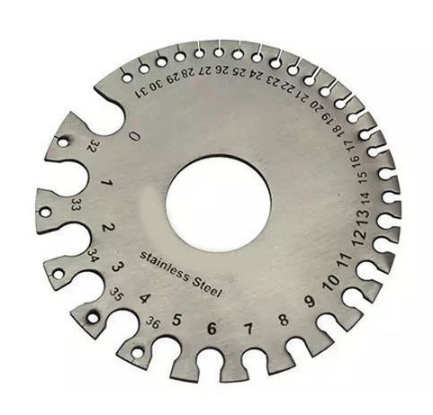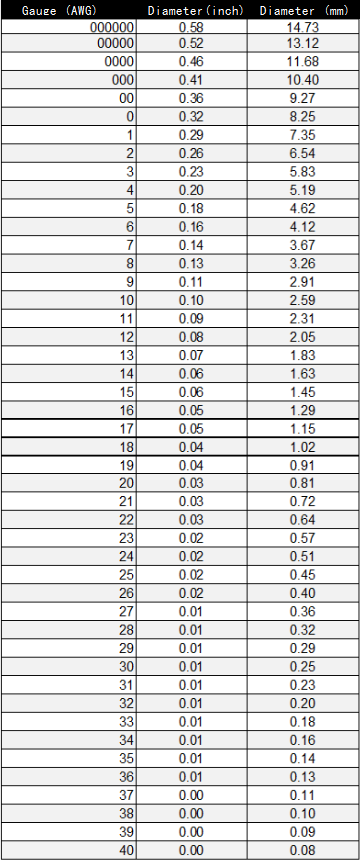> > Wire Gauge Chart & Calculations | What is American Wire Gauge | CNCLATHING
> > Wire Gauge Chart & Calculations | What is American Wire Gauge | CNCLATHING

# Wire Gauge Chart & Calculations | What is American Wire Gauge | CNCLATHING

2020.3.23

Wire gauge is a measurement system of the wire size, it determines the amount of electric current a wire can safely carry, as well as its electrical resistance and weight. The development of standardized wire gauges helps the selection of the right wires for particular purposes. Here is the introduction to American Wire Gauge and AWG chart (wire gauge chart) with detailed data for reference and download.#### What is American Wire Gauge?

American Wire Gauge (AWG), is a logarithmic stepped standardized wire gauge system used for the diameters of round, solid, nonferrous, electrically conducting wire, which also can be used to specify body piercing jewelry sizes, even for non-metallic materials. The larger gauge number in the AWG wire size chart indicates smaller wire diameters. This wire gauge sizes system originated in the number of drawing operations used to produce a given gauge of wire. The finer wire needs more drawing. Very fine wire (for example, 30 gauge) required more passes through the drawing dies than 0 gauge wire did. In the AWG gauge system, the diameter of the wire is doubled for every six wire gauges, and the cross-sectional area of the wire is doubled for every three wire gauges. In the American Wire Gauge (AWG) system, wire size diameters can be calculated by applying the formula D(AWG)=.005·92((36-AWG)/39) inch.

## Wire Gauge Calculation Formula

Wire diameter:

dn (in) = 0.005 in × 92(36-n)/39

dn (mm) = 0.127 mm × 92(36-n)/39

Wire cross-sectional area:

An (kcmil) = 1000×dn2 = 0.025 in2 × 92(36-n)/19.5

An (in2) = (π/4)×dn2 = 0.000019635 in2 × 92(36-n)/19.5

An (mm2) = (π/4)×dn2 = 0.012668 mm2 × 92(36-n)/19.5

Wire resistance:

R(Ω/kft) = 0.3048 × 109 × ρ(Ω·m) / (25.42 × An (in2))

R(Ω/km) = 109 × ρ(Ω·m) / An (mm2)

#### Wire Gauge Chart - AWG Chart

Here is the wire size chart, also known as AWG chart or wire diameter chart, applicable for a single, solid, and round conductor. The gauge of stranded wire is determined by the cross-sectional area of the equivalent solid conductor. The American Wire Gauge Chart shows wire gauge diameter in inches (in) and millimeters (mm). You can use it to convert AWG to mm or inch.